Successfully reported this slideshow.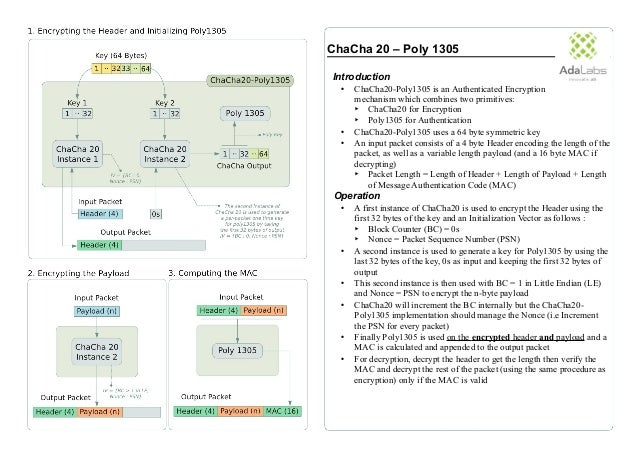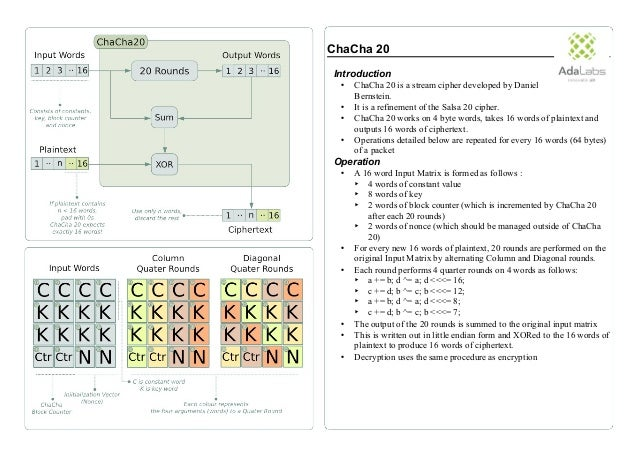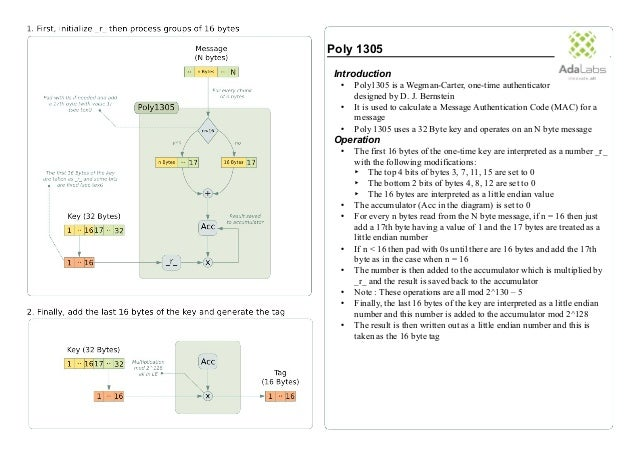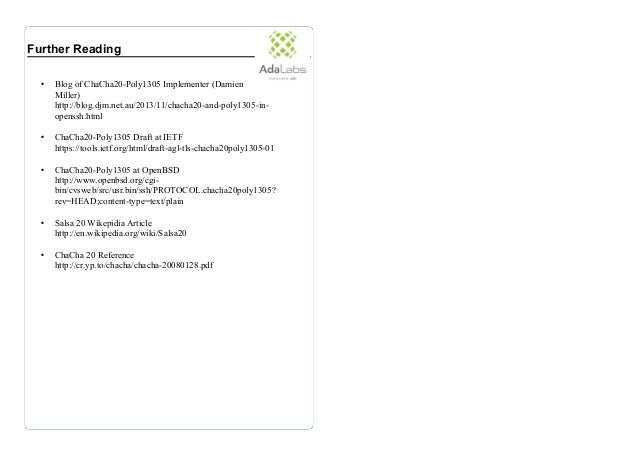Upcoming SlideShare
×

• Full Name
Comment goes here.

Are you sure you want to Yes No• Be the first to comment

1. 1. ChaCha 20 – Poly 1305 Introduction • ChaCha20-Poly1305 is an Authenticated Encryption mechanism which combines two primitives: ‣ ChaCha20 for Encryption ‣ Poly1305 for Authentication • ChaCha20-Poly1305 uses a 64 byte symmetric key • An input packet consists of a 4 byte Header encoding the length of the packet, as well as a variable length payload (and a 16 byte MAC if decrypting) ‣ Packet Length = Length of Header + Length of Payload + Length of Message Authentication Code (MAC) Operation • A first instance of ChaCha20 is used to encrypt the Header using the first 32 bytes of the key and an Initialization Vector as follows : ‣ Block Counter (BC) = 0s ‣ Nonce = Packet Sequence Number (PSN) • A second instance is used to generate a key for Poly1305 by using the last 32 bytes of the key, 0s as input and keeping the first 32 bytes of output • This second instance is then used with BC = 1 in Little Endian (LE) and Nonce = PSN to encrypt the n-byte payload • ChaCha20 will increment the BC internally but the ChaCha20- Poly1305 implementation should manage the Nonce (i.e Increment the PSN for every packet) • Finally Poly1305 is used on the encrypted header and payload and a MAC is calculated and appended to the output packet • For decryption, decrypt the header to get the length then verify the MAC and decrypt the rest of the packet (using the same procedure as encryption) only if the MAC is valid
2. 2. ChaCha 20 Introduction • ChaCha 20 is a stream cipher developed by Daniel Bernstein. • It is a refinement of the Salsa 20 cipher. • ChaCha 20 works on 4 byte words, takes 16 words of plaintext and outputs 16 words of ciphertext. • Operations detailed below are repeated for every 16 words (64 bytes) of a packet Operation • A 16 word Input Matrix is formed as follows : ‣ 4 words of constant value ‣ 8 words of key ‣ 2 words of block counter (which is incremented by ChaCha 20 after each 20 rounds) ‣ 2 words of nonce (which should be managed outside of ChaCha 20) • For every new 16 words of plaintext, 20 rounds are performed on the original Input Matrix by alternating Column and Diagonal rounds. • Each round performs 4 quarter rounds on 4 words as follows: ‣ a += b; d ^= a; d <<<= 16; ‣ c += d; b ^= c; b <<<= 12; ‣ a += b; d ^= a; d <<<= 8; ‣ c += d; b ^= c; b <<<= 7; • The output of the 20 rounds is summed to the original input matrix • This is written out in little endian form and XORed to the 16 words of plaintext to produce 16 words of ciphertext. • Decryption uses the same procedure as encryption
3. 3. Poly 1305 Introduction • Poly1305 is a Wegman-Carter, one-time authenticator designed by D. J. Bernstein • It is used to calculate a Message Authentication Code (MAC) for a message • Poly 1305 uses a 32 Byte key and operates on an N byte message Operation • The first 16 bytes of the one-time key are interpreted as a number _r_ with the following modifications: ‣ The top 4 bits of bytes 3, 7, 11, 15 are set to 0 ‣ The bottom 2 bits of bytes 4, 8, 12 are set to 0 ‣ The 16 bytes are interpreted as a little endian value • The accumulator (Acc in the diagram) is set to 0 • For every n bytes read from the N byte message, if n = 16 then just add a 17th byte having a value of 1 and the 17 bytes are treated as a little endian number • If n < 16 then pad with 0s until there are 16 bytes and add the 17th byte as in the case when n = 16 • The number is then added to the accumulator which is multiplied by _r_ and the result is saved back to the accumulator • Note : These operations are all mod 2^130 – 5 • Finally, the last 16 bytes of the key are interpreted as a little endian number and this number is added to the accumulator mod 2^128 • The result is then written out as a little endian number and this is taken as the 16 byte tag
4. 4. Further Reading • Blog of ChaCha20-Poly1305 Implementer (Damien Miller) http://blog.djm.net.au/2013/11/chacha20-and-poly1305-in- openssh.html • ChaCha20-Poly1305 Draft at IETF https://tools.ietf.org/html/draft-agl-tls-chacha20poly1305-01 • ChaCha20-Poly1305 at OpenBSD http://www.openbsd.org/cgi- bin/cvsweb/src/usr.bin/ssh/PROTOCOL.chacha20poly1305? rev=HEAD;content-type=text/plain • Salsa 20 Wikepidia Article http://en.wikipedia.org/wiki/Salsa20 • ChaCha 20 Reference http://cr.yp.to/chacha/chacha-20080128.pdf

### Be the first to comment

•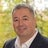#### romain_jacotin

Apr. 30, 2015
•#### tobeand

Sep. 9, 2015
•#### sangsoo_hong

Oct. 2, 2015
•#### selvagangai

Sep. 16, 2019

Total views

6,955

On Slideshare

0

From embeds

0

Number of embeds

3,568

77

Shares

0